# Top 100 R Interview Questions and Answers for 2022

R is one of the most popular programming language for performing statistical analysis and predictive modeling. Many recent surveys and studies claimed "R" holds a good percentage of market share in analytics industry. Data scientist role generally requires a candidate to know R/Python programming language. People who know R programming language are generally paid more than python and SAS programmers. In terms of advancement in R software, it has improved a lot in the recent years. It supports parallel computing and integration with big data technologies.R Interview Questions and Answers

The following is a list of most frequently asked R Programming Interview Questions with detailed answer. It includes some basic, advanced or tricky questions related to R. Also it covers interview questions related to data science with R.

1. How to determine data type of an object?

class() is used to determine data type of an object. See the example below -
x <- factor(1:5)
class(x)
It returns factor.Object Class
To determine structure of an object, use str() function :
str(x) returns "Factor w/ 5 level"

Example 2 :
xx <- data.frame(var1=c(1:5))
class(xx)
It returns "data.frame".
str(xx) returns 'data.frame' : 5 obs. of  1 variable: \$ var1: int

2.  What is the use of mode() function?

It returns the storage mode of an object.
x <- factor(1:5)
mode(x)
The above mode function returns numeric.Mode Function
x <- data.frame(var1=c(1:5))
mode(x)
It returns list.

3. Which data structure is used to store categorical variables?

R has a special data structure called "factor" to store categorical variables. It tells R that a variable is nominal or ordinal by making it a factor.
gender = c(1,2,1,2,1,2)
gender = factor(gender)
gender
4. How to check the frequency distribution of a categorical variable?

The table function is used to calculate the count of each categories of a categorical variable.
gender = factor(c("m","f","f","m","f","f"))
table(gender)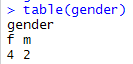Output

If you want to include % of values in each group, you can store the result in data frame using data.frame function and the calculate the column percent.
t = data.frame(table(gender))
t\$percent= round(t\$Freq / sum(t\$Freq)*100,2)Frequency Distribution

5.  How to check the cumulative frequency distribution of a categorical variable

The cumsum function is used to calculate the cumulative sum of a categorical variable.
gender = factor(c("m","f","f","m","f","f"))
x = table(gender)
cumsum(x)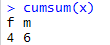Cumulative Sum

If you want to see the cumulative percentage of values, see the code below :
t = data.frame(table(gender))
t\$cumfreq = cumsum(t\$Freq)
t\$cumpercent= round(t\$cumfreq / sum(t\$Freq)*100,2)Cumulative Frequency Distribution

6. How to produce histogram

The hist function is used to produce the histogram of a variable.
df = sample(1:100, 25)
hist(df, right=FALSE)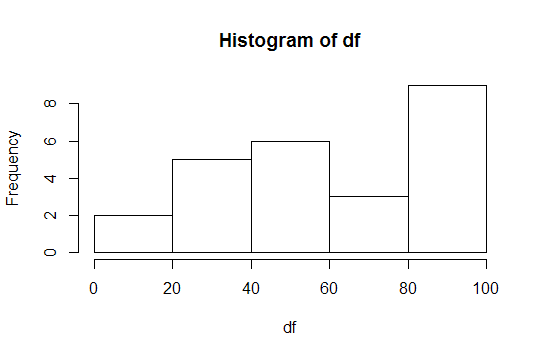Produce Histogram with R
To improve the layout of histogram, you can use the code below
colors = c("red", "yellow", "green", "violet", "orange", "blue", "pink", "cyan")
hist(df,  right=FALSE,  col=colors, main="Main Title ", xlab="X-Axis Title")
7. How to produce bar graph

First calculate the frequency distribution with table function and then apply barplot function to produce bar graph
mydata = sample(LETTERS[1:5],16,replace = TRUE)
mydata.count= table(mydata)
barplot(mydata.count)
To improve the layout of bar graph, you can use the code below:
colors = c("red", "yellow", "green", "violet", "orange", "blue", "pink", "cyan")
barplot(mydata.count, col=colors, main="Main Title ", xlab="X-Axis Title")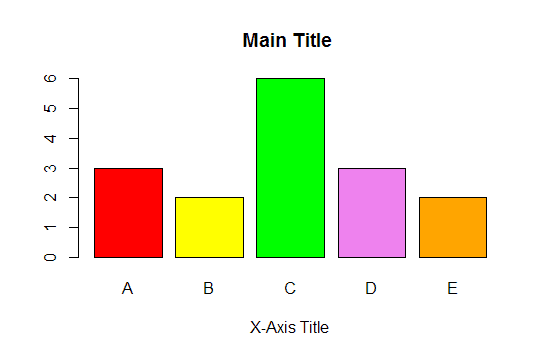Bar Graph with R

8. How to produce Pie Chart

First calculate the frequency distribution with table function and then apply pie function to produce pie chart.
mydata = sample(LETTERS[1:5],16,replace = TRUE)
mydata.count= table(mydata)
pie(mydata.count, col=rainbow(12))Pie Chart with R

9. Multiplication of 2 vectors having different length

For example, you have two vectors as defined below -
x <- c(4,5,6)
y <- c(2,3)
If you run this vector z <- x*y , what would be the output? What would be the length of z?

It returns 8 15 12 with the warning message as shown below. The length of z is 3 as it has three elements.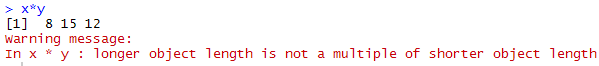Multiplication of vectors

First Step : It performs multiplication of the first element of vector x i.e. 4 with first element of vector y i.e. 2 and the result is 8. In the second step, it multiplies second element of vector x i.e. 5 with second element of vector b i.e. 3, and the result is 15. In the next step, R multiplies first element of smaller vector (y) with last element of bigger vector x.

Suppose the vector x would contain four elements as shown below :
x <- c(4,5,6,7)
y <- c(2,3)
x*y
It returns 8 15 12 21. It works like this : (4*2) (5*3) (6*2) (7*3)

10. What are the different data structures R contain?

R contains primarily the following data structures :
1. Vector
2. Matrix
3. Array
4. List
5. Data frame
6. Factor
The first three data types (vector, matrix, array) are homogeneous in behavior. It means all contents must be of the same type. The fourth and fifth data types (list, data frame) are heterogeneous in behavior. It implies they allow different types. And the factor data type is used to store categorical variable.

11. How to combine data frames?

Let's prepare 2 vectors for demonstration :
x = c(1:5)
y = c("m","f","f","m","f")
The cbind() function is used to combine data frame by columns.
z=cbind(x,y)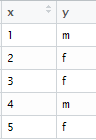cbind : Output

The rbind() function is used to combine data frame by rows.
z = rbind(x,y)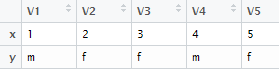rbind : Output

While using cbind() function, make sure the number of rows must be equal in both the datasets. While using rbind() function, make sure both the number and names of columns must be same. If names of columns would not be same, wrong data would be appended to columns or records might go missing.

12. How to combine data by rows when different number of columns?

When the number of columns in datasets are not equal, rbind() function doesn't work to combine data by rows. For example, we have two data frames df and df2. The data frame df has 2 columns and df2 has only 1 variable. See the code below -
df = data.frame(x = c(1:4), y = c("m","f","f","m"))
df2 = data.frame(x = c(5:8))
The bind_rows() function from dplyr package can be used to combine data frames when number of columns do not match.
library(dplyr)
combdf = bind_rows(df,df2)

Tutorial : dplyr Tutorial with 50 Examples

13.  What are valid variable names in R?

A valid variable name consists of letters, numbers and the dot or underline characters. A variable name can start with either a letter or the dot followed by a character (not number).
A variable name such as .1var is not valid. But .var1 is valid.
A variable name cannot have reserved words. The reserved words are listed below -
if else repeat while function for in next break
TRUE FALSE NULL Inf NaN NA NA_integer_ NA_real_ NA_complex_ NA_character_
A variable name can have maximum to 10,000 bytes.

14. What is the use of with() and by() functions? What are its alternatives?

Suppose you have a data frame as shown below -
df=data.frame(x=c(1:6), y=c(1,2,4,6,8,12))
You are asked to perform this calculation : (x+y) + (x-y) . Most of the R programmers write like code below -
(df\$x + df\$y) + (df\$x - df\$y)
Using with() function, you can refer your data frame and make the above code compact and simpler-
with(df, (x+y) + (x-y))
The with() function is equivalent to pipe operator in dplyr package. See the code below -
library(dplyr)
df %>% mutate((x+y) + (x-y))

by() function in R

The by() function is equivalent to group by function in SQL. It is used to perform calculation by a factor or a categorical variable. In the example below, we are computing mean of variable var2 by a factor var1.
df = data.frame(var1=factor(c(1,2,1,2,1,2)), var2=c(10:15))
with(df, by(df, var1, function(x) mean(x\$var2)))
The group_by() function in dply package can perform the same task.
library(dplyr)
df %>% group_by(var1)%>% summarise(mean(var2))

15. How to rename a variable?

In the example below, we are renaming variable var1 to variable1.
df = data.frame(var1=c(1:5))
colnames(df)[colnames(df) == 'var1'] <- 'variable1'
The rename() function in dplyr package can also be used to rename a variable.
library(dplyr)
df= rename(df, variable1=var1)

16. What is the use of which() function in R?

The which() function returns the position of elements of a logical vector that are TRUE. In the example below, we are figuring out the row number wherein the maximum value of a variable x is recorded.
mydata=data.frame(x = c(1,3,10,5,7))
which(mydata\$x==max(mydata\$x))
It returns 3 as 10 is the maximum value and it is at 3rd row in the variable x.

17. How to calculate first non-missing value in variables?

Suppose you have three variables X, Y and Z and you need to extract first non-missing value in each rows of these variables.
X Y Z
NA 1 5
3 NA 2
The coalesce() function in dplyr package can be used to accomplish this task.
library(dplyr)
data %>% mutate(var=coalesce(X,Y,Z))COALESCE Function in R

18. How to calculate max value for rows?

Let's create a sample data frame
X Y Z
7 NA 5
2 4 5
With apply() function, we can tell R to apply the max function rowwise. The na,rm = TRUE is used to tell R to ignore missing values while calculating max value. If it is not used, it would return NA.
dt1\$var = apply(dt1,1, function(x) max(x,na.rm = TRUE))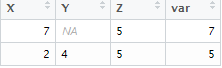Output

19. Count number of zeros in a row
A B C
8 0 0
6 0 5
apply(dt2,1, function(x) sum(x==0))

20. Does the following code work?
ifelse(df\$var1==NA, 0,1)
It does not work. The logic operation on NA returns NA. It does not TRUE or FALSE.

This code works ifelse(is.na(df\$var1), 0,1)

21. What would be the final value of x after running the following program?

x = 3
mult <- function(j)
{
x = j * 2
return(x)
}

mult(2)
 4

Answer : The value of 'x' will remain 3. See the output shown in the image below-Output
It is because x is defined outside function. If you want to change the value of x after running the function, you can use the following program:
x = 3
mult <- function(j)
{
x <<- j * 2
return(x)
}
mult(2)
x
The operator "<<-" tells R to search in the parent environment for an existing definition of the variable we want to be assigned.

22. How to convert a factor variable to numeric

The as.numeric() function returns a vector of the levels of your factor and not the original values. Hence, it is required to convert a factor variable to character before converting it to numeric.
a <- factor(c(5, 6, 7, 7, 5))
a1 = as.numeric(as.character(a))
23. How to concatenate two strings?

The paste() function is used to join two strings. A single space is the default separator between two strings.
a = "Deepanshu"
b = "Bhalla"
paste(a, b)
It returns "Deepanshu Bhalla"

If you want to change the default single space separator, you can add sep="," keyword to include comma as a separator.
paste(a, b, sep=",") returns "Deepanshu,Bhalla"

24. How to extract first 3 characters from a word

The substr() function is used to extract strings in a character vector. The syntax of substr function is substr(character_vector, starting_position, end_position)
x = "AXZ2016"
substr(x,1,3)
Character Functions Explained

25. How to extract last name from full name

The last name is the end string of the name. For example, Jhonson is the last name of "Dave,Jon,Jhonson".
var
Sandy,Jones
Dave,Jon,Jhonson
The word() function of stringr package is used to extract or scan word from a string. -1 in the second parameter  denotes the last word.
library(stringr)
dt2\$var2 = word(dt2\$var, -1, sep = ",")

26. How to remove leading and trailing spaces

The trimws() function is used to remove leading and trailing spaces.
a = " David Banes "
trimws(a)
It returns "David Banes".

27. How to generate random numbers between 1 and 100

The runif() function is used to generate random numbers.
rand = runif(100, min = 1, max = 100)

28. How to apply LEFT JOIN in R?

LEFT JOIN implies keeping all rows from the left table (data frame) with the matches rows from the right table. In the merge() function, all.x=TRUE denotes left join.
df1=data.frame(ID=c(1:5), Score=runif(5,50,100))
df2=data.frame(ID=c(3,5,7:9), Score2=runif(5,1,100))
comb = merge(df1, df2, by ="ID", all.x = TRUE)
Left Join (SQL Style)
library(sqldf)
comb = sqldf('select df1.*, df2.* from df1 left join df2 on df1.ID = df2.ID')

Left Join with dply package
library(dplyr)
comb = left_join(df1, df2, by = "ID")

29. How to calculate cartesian product of two datasets

The cartesian product implies cross product of two tables (data frames). For example, df1 has 5 rows and df2 has 5 rows. The combined table would contain 25 rows (5*5)
comb = merge(df1,df2,by=NULL)
CROSS JOIN (SQL Style)
library(sqldf)
comb2 = sqldf('select * from df1 join df2 ')

30. Unique rows common to both the datasets

First, create two sample data frames

df1=data.frame(ID=c(1:5), Score=c(50:54))
df2=data.frame(ID=c(3,5,7:9), Score=c(52,60:63))
library(dplyr)
comb = intersect(df1,df2)
library(sqldf)
comb2 = sqldf('select * from df1 intersect select * from df2 ')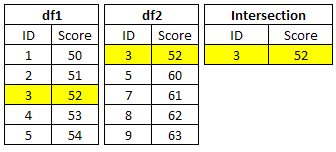Output : Intersection with R

31. How to measure execution time of a program in R?

There are multiple ways to measure running time of code. Some frequently used methods are listed below -

R Base Method
start.time <- Sys.time()
runif(5555,1,1000)
end.time <- Sys.time()
end.time - start.time
With tictoc package
library(tictoc)
tic()
runif(5555,1,1000)
toc()

32. Which package is generally used for fast data manipulation on large datasets?

The package data.table performs fast data manipulation on large datasets. See the comparison between dplyr and data.table.

library(nycflights13)
data(flights)
df = setDT(flights)

# Load required packages
library(tictoc)
library(dplyr)
library(data.table)

# Using data.table package
tic()
df[arr_delay > 30 & dest == "IAH",
.(avg = mean(arr_delay),
size = .N),
by = carrier]
toc()

# Using dplyr package
tic()
flights %>% filter(arr_delay > 30 & dest == "IAH") %>%
group_by(carrier) %>% summarise(avg = mean(arr_delay), size = n())
toc()

Result : data.table package took 0.04 seconds. whereas dplyr package took 0.07 seconds. So, data.table is approx. 40% faster than dplyr. Since the dataset used in the example is of medium size, there is no noticeable difference between the two. As size of data grows, the difference of execution time gets bigger.

33. How to read large CSV file in R?

We can use fread() function of data.table package.
library(data.table)

We can also use read.big.matrix() function of bigmemory package.

34. What is the difference between the following two programs ?

1. temp = data.frame(v1<-c(1:10),v2<-c(5:14))
2. temp = data.frame(v1=c(1:10),v2=c(5:14))

In the first case, it created two vectors v1 and v2 and a data frame temp which has 2 variables with improper variable names. The second code creates a data frame temp with proper variable names.

35. How to remove all the objects
rm(list=ls())

36. What are the various sorting algorithms in R?

Major five sorting algorithms :
1. Bubble Sort
2. Selection Sort
3. Merge Sort
4. Quick Sort
5. Bucket Sort

37. Sort data by multiple variables

Create a sample data frame
mydata = data.frame(score = ifelse(sign(rnorm(25))==-1,1,2),
experience= sample(1:25))
Task : You need to sort score variable on ascending order and then sort experience variable on descending order.

R Base Method
mydata1 <- mydata[order(mydata\$score, -mydata\$experience),]
With dplyr package
library(dplyr)
mydata1 = arrange(mydata, score, desc(experience))

38. Drop Multiple Variables

Suppose you need to remove 3 variables - x, y and z from data frame "mydata".

R Base Method
df = subset(mydata, select = -c(x,y,z))
With dplyr package
library(dplyr)
df = select(mydata, -c(x,y,z))

40. How to save everything in R session
save.image(file="dt.RData")

41. How R handles missing values?

Missing values are represented by capital NA.

To create a new data without any missing value, you can use the code below :
df <- na.omit(mydata)

42. How to remove duplicate values by a column

Suppose you have a data consisting of 25 records. You are asked to remove duplicates based on a column. In the example, we are eliminating duplicates by variable y.
data = data.frame(y=sample(1:25, replace = TRUE), x=rnorm(25))
R Base Method
test = subset(data, !duplicated(data[,"y"]))
dplyr Method
library(dplyr)
test1 = distinct(data, y, .keep_all= TRUE)

43. Which packages are used for transposing data with R

The reshape2 and tidyr packages are most popular packages for reshaping data in R.

Explanation : Transpose Data

44. Calculate number of hours, days, weeks, months and years between 2 dates

Let's set 2 dates :
dates <- as.Date(c("2015-09-02", "2016-09-05"))
difftime(dates, dates, units = "hours")
difftime(dates, dates, units = "days")
floor(difftime(dates, dates, units = "weeks"))
floor(difftime(dates, dates, units = "days")/365)
With lubridate package
library(lubridate)
interval(dates, dates) %/% hours(1)
interval(dates, dates) %/% days(1)
interval(dates, dates) %/% weeks(1)
interval(dates, dates) %/% months(1)
interval(dates, dates) %/% years(1)
The number of months unit is not included in the base difftime() function so we can use interval() function of lubridate() package.

45. How to add 3 months to a date
mydate <- as.Date("2015-09-02")
mydate + months(3)

46. Extract date and time from timestamp
mydate <- as.POSIXlt("2015-09-27 12:02:14")
library(lubridate)
date(mydate) # Extracting date part
format(mydate, format="%H:%M:%S") # Extracting time part
Extracting various time periods
day(mydate)
month(mydate)
year(mydate)
hour(mydate)
minute(mydate)
second(mydate)

47. What are various ways to write loop in R

There are primarily three ways to write loop in R
1. For Loop
2. While Loop
3. Apply Family of Functions such as Apply, Lapply, Sapply etc

48. Difference between lapply and sapply in R

lapply returns a list when we apply a function to each element of a data structure. whereas sapply returns a vector.

49. Difference between sort(), rank() and order() functions?

The sort() function is used to sort a 1 dimension vector or a single variable of data.
The rank() function returns the ranking of each value.
The order() function returns the indices that can be used to sort the data.

Example :
set.seed(1234)
x = sample(1:50, 10)
x
  6 31 30 48 40 29  1 10 28 22

sort(x)
  1  6 10 22 28 29 30 31 40 48

It sorts the data on ascending order.

rank(x)
  2  8  7 10  9  6  1  3  5  4

2 implies the number in the first position is the second lowest and 8 implies the number in the second position is the eighth lowest.

order(x)
  7  1  8 10  9  6  3  2  5  4

7 implies the 7th value of x is the smallest value, so 7 is the first element of order(x) and i refers to the first value of x is the second smallest.
If you run x[order(x)], it would give you the same result as sort() function. The difference between these two functions lies in two or more dimensions of data (two or more columns). In other words, the sort() function cannot be used for more than 1 dimension whereas x[order(x)] can be used.

50.  Extracting Numeric Variables
cols <- sapply(mydata, is.numeric)
abc = mydata [,cols]

Data Science with R Interview Questions

The list below contains most frequently asked interview questions for a role of data scientist. Most of the roles related to data science or predictive modeling require candidate to be well conversant with R and know how to develop and validate predictive models with R.

51. Which function is used for building linear regression model?

The lm() function is used for fitting a linear regression model.

52. How to add interaction in the linear regression model?

:An interaction can be created using colon sign (:). For example, x1 and x2 are two predictors (independent variables). The interaction between the variables can be formed like x1:x2.
See the example below -
linreg1 <- lm(y ~ x1 + x2 + x1:x2, data=mydata)
The above code is equivalent to the following code :
linreg1 <- lm(y ~ x1*x2, data=mydata)
x1:x2 - It implies including both main effects (x1 + x2) and interaction (x1:x2).

53. How to check autocorrelation assumption for linear regression?

durbinWatsonTest() function

54. Which function is useful for developing a binary logistic regression model?

glm() function with family = "binomial"

55. How to perform stepwise variable selection in logistic regression model?

Run step() function after building logistic model with glm() function.

56. How to do scoring in the logistic regression model?

Run predict(logit_model, validation_data, type = "response")

57. How to split data into training and validation?
dt = sort(sample(nrow(mydata), nrow(mydata)*.7))
train<-mydata[dt,]
val<-mydata[-dt,]

58. How to standardize variables?

data2 = scale(data)

59. How to validate cluster analysis

Validate Cluster Analysis

60. Which are the popular R packages for decision tree?

rpart, party

61. What is the difference between rpart and party package for developing a decision tree model?

rpart is based on Gini Index which measures impurity in node. Whereas ctree() function from "party" package uses a significance test procedure in order to select variables.

62. How to check correlation with R?

cor() function

63. Have you heard 'relaimpo' package?

It is used to measure the relative importance of independent variables in a model.

64. How to fine tune random forest model?

Use tuneRF() function

65. What shrinkage defines in gradient boosting model?

Shrinkage is used for reducing, or shrinking, the impact of each additional fitted base-learner (tree).

66. How to make data stationary for ARIMA time series model?

Use ndiffs() function which returns the number of difference required to make data stationary.

67. How to automate arima model?

Use auto.arima() function of forecast package

68. How to fit proportional hazards model in R?

Use coxph() function of survival package.

69. Which package is used for market basket analysis?

arules package

70. Parallelizing Machine Learning Algorithms

Link : Parallelizing Machine LearningShare
Related PostsDeepanshu founded ListenData with a simple objective - Make analytics easy to understand and follow. He has over 10 years of experience in data science. During his tenure, he has worked with global clients in various domains like Banking, Insurance, Private Equity, Telecom and Human Resource.

14 Responses to "Top 100 R Interview Questions and Answers for 2022"
1.This is a really interesting post. It is useful for more than just interview questions. It is more like insights into how R works.

1.Thank you for stopping by my blog. Cheers!

2.Agree with Mike, above this is a neat compendium on R features and tips & tricks to handle data. Well done Deepanshu.

1.Thank you for your lovely words!

3.Awesome!

4.I see many times refering to dplyr package. How relevant may it be? I never use it, there is nothing on dplyr that I cannot do with R-base functions, and I dont like it...

5.This is really good deepanshu.

6.please give some tutorial on data visulization also

7.Thank you for sharing great list of data science interview questions and answers. I am looking for more question and answer.

8.Thank you sir it's amazing tutorial for freshers and experienced

9.Thanks Deepanshu for sharing very informative and useful questionnaire.

10.Very Informative ..Done decent job

11.Very useful information Thanks

12.I learned World's Trending Technology from certified experts for free of cost. I Got a job in decent Top MNC Company with handsome 14 LPA salary, I have learned the World's Trending Technology from python training in btm layout experts who know advanced concepts which can help to solve any type of Real-time issues in the field of Python. Really worth trying instant approval blog commenting sites

Next → ← Prev